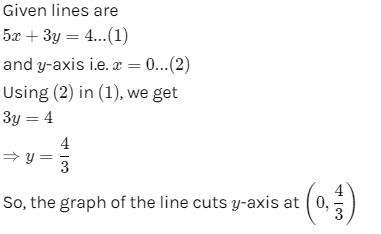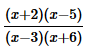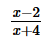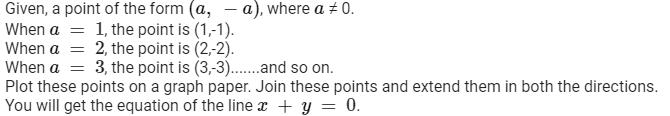# Test: Linear Equations In Two Variables - 1

## 25 Questions MCQ Test Mathematics (Maths) Class 9 | Test: Linear Equations In Two Variables - 1

Description
This mock test of Test: Linear Equations In Two Variables - 1 for Class 9 helps you for every Class 9 entrance exam. This contains 25 Multiple Choice Questions for Class 9 Test: Linear Equations In Two Variables - 1 (mcq) to study with solutions a complete question bank. The solved questions answers in this Test: Linear Equations In Two Variables - 1 quiz give you a good mix of easy questions and tough questions. Class 9 students definitely take this Test: Linear Equations In Two Variables - 1 exercise for a better result in the exam. You can find other Test: Linear Equations In Two Variables - 1 extra questions, long questions & short questions for Class 9 on EduRev as well by searching above.
QUESTION: 1

Solution:
QUESTION: 2

Solution:
QUESTION: 3

### The graph of the equation 2x – 3 = 3x – 5 is parallel to :

Solution:
QUESTION: 4

The straight line 2x – 5y = 0 passes through the point :

Solution:
QUESTION: 5

The graph of the lines x + y = 7 and x – y = 3 meet at the point :

Solution:
QUESTION: 6

The graph of the equation y = x2 is :

Solution:

In the graph of y = x2, the point (0, 0) is called the vertex. The vertex is the minimum point in a parabola that opens upward. In a parabola that opens downward, the vertex is the maximum point. We can graph a parabola with a different vertex.

QUESTION: 7

Which of the following equations is not linear equation :

Solution:
QUESTION: 8

If x = 1,y = 1 is a solution of equation 9ax + 12ay = 63 then, the value of a is :

Solution:
QUESTION: 9

The graph of the line 5x + 3y = 4 cuts y-axis at the point :

Solution:QUESTION: 10

A linear equation in two variables has maximum :

Solution:
QUESTION: 11

The equation=has

Solution:
QUESTION: 12

If x = a, y = b is the solution of the pair of equation x-y = 2 and x+y = 4 then what will be value of a and b

Solution:
QUESTION: 13

The solution of the equation x + y = 3, 3x – 2y = 4 is :

Solution:
QUESTION: 14

The value of x satisfying the equation x2 + p2 = (q – x)2 is :

Solution:

Solution :

The correct option is Option B.

n² + p² = (q + n)²

n² + p² = q² - 2qn + n²

Eliminating n² from both sides,

p² = q² - 2qn

n = q² - p² / 2q

QUESTION: 15

The sum of two digits and the number formed by interchanging its digit is 110. If ten is subtracted from the first number, the new number is 4 more than 5 times of the sum of the digits in the first number. Find the first number.

Solution:

let the unit place digit be x and tens place digit be y

then the two-digit number will be  10y + x

and the number formed by interchanging the unit place and tens place digits will be 10x + y

according to the first condition given in the qs i.e, the sum of two numbers is 110 that  is

10y + x + 10x + y = 110

=> 11x + 11y = 110

divide the above equation by 11 we get

x + y = 10

x = 10 - y ....(i)

now according to the second equation,

if 10 is subtracted from the first number i.e, the new number is  10y + x - 10

given that the new number is 4 more than 5 time the sum of its digits in the first number i.e

the sum of its digits in the first number is  x + y,  now 5 times of its, 5(x + y), and now 4 more that is, 4 + 5(x + y)

therefore new number = 4 + 5(x + y)

10y + x - 10 = 4 +5(x + y)

10y - 5y + x = 4 +10 +5x

5y = 14 + 4x.....(ii)

substitute the value of x from eq(i) to eq (ii)

we  get , 5y = 14 + 4(10 - y)

5y = 14 + 40 - 4y

y = 6

and from eq(i)

x = 4

then the first number 10y + x = 10x6 + 4 = 64

first number is 64.

QUESTION: 16

x = 0 is the equation of

Solution:
QUESTION: 17

y = 0 is the equation of

Solution:
QUESTION: 18

x – 4 is the equation of

Solution:
QUESTION: 19

y + 7 is the equation of

Solution:
QUESTION: 20

The point of the form (a, -a), where  a ≠ 0, lies on

Solution:QUESTION: 21

The point of the form (a, -a), where a , 0 lies on

Solution:
QUESTION: 22

The point of the form (-a, a), where a , 0 lies on

Solution:
QUESTION: 23

The linear equation 2x + 3y = 6 has

Solution:
QUESTION: 24

The graph of the linear equation 2x – 3y = 6, cuts the y-axis at the point

Solution:
QUESTION: 25

The graph of the linear equation 3x – 2y = 6, cuts the x-axis at the point

Solution: